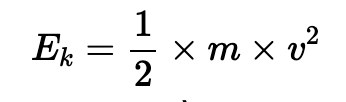# Help Zone

### Student Question

What is the kinetic energy calculation

Science{t c="richEditor.description.title"} {t c="richEditor.description.paragraphMenu"} {t c="richEditor.description.inlineMenu"} {t c="richEditor.description.embed"}

## Explanations (1)

•Explanation from AlloprofExplanation from Alloprof

This Explanation was submitted by a member of the Alloprof team.

Hello PhilosophicalAnt2817,

Thanks for reaching out!

Kinetic energy is the energy of motion. Therefore, in order to calculate it, we must consider the mass and the speed of the object.

Therefore, the formula is the following :where Ek = kinetic energy (J), m = mass (kg) and v = speed (m/s)

Therefore, if an object goes at the same speed but we double its mass, its kinetic energy will double as well.

Reach out if there's anything else!

Emilie